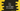# C++ program to find the sum of digits of a number### C++ program to find the sum of digits of a number:

In this post, we will learn how to find the sum of all digits of a number in C++. Our program will take one number as input from the user, calculate the sum of its digits and print it out. We will use one while loop to find the digits of the given number and use one variable to store the sum of all the digits. With this program, you will learn how we can use find all digits of a number and how we can use for loop to calculate something in C++.

### Algorithm to use:

We will use the below steps for this program:

1. Get the number from the user
2. Use one loop and pick the digits of the number one by one. Keep adding these digits to a variable to calculate the sum. Change the number to number/10
3. Print out the sum.

That’s all.

To get the last digit of a number, we will use modulo or %. For example, for the number 123:

• Current value 123. Get the last digit: 123%10 = 3. Change it to 123/10 = 12. Total sum 0+3 = 3
• Current value 12. Get the last digit: 12%10 = 2. Change it to 12/10 = 1. Total sum 3+2 = 5
• Current value 1. Total sum 5+1 = 6

### C++ program:

Below is the complete C++ program:

``````#include <iostream>

using namespace std;

int main()
{
int givenNumber;
int sum = 0;

cout << "Enter a number :" << endl;
cin >> givenNumber;

while (givenNumber > 0)
{
sum += givenNumber % 10;
givenNumber = givenNumber / 10;
}

cout << "Total sum of digits :" << sum << endl;
}``````

### Explanation:

1. givenNumber is an integer variable to hold the user input number.
2. sum variable is initialized as 0 to hold the sum of digits.
3. We are reading the number as user input using cin.
4. Using a while loop, we are finding the last digit of the number and adding it to the variable sum. This loop will run till the value of givenNumber is greater than 0. We are also changing its value to givenNumber / 10 on each iteration.
5. After the while loop ends, we are printing the value of the sum.

### Sample Output:

``````Enter a number :
123
Total sum of digits :6

Enter a number :
23345
Total sum of digits :17``````